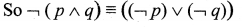## Tamilnadu Samacheer Kalvi 12th Maths Solutions Chapter 12 Discrete Mathematics Ex 12.2

Question 1.
Let p : Jupiter is a planet and q : India is an island be any two simple statements. Give verbal sentence describing each of the following statements.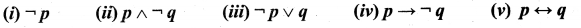Solution:
(i) $$\neg p$$ : Jupiter is not a planet
(ii) $$p \wedge \neg q$$ : Jupiter is not a planet and India is not an island
(iii) $$\neg p \vee q$$ : Jupiter is not a planet or India is an island.
(iv) $$p \rightarrow \neg q$$ : If Jupiter is a planet then India is not an island
(v) $$p \leftrightarrow q$$ : If Jupiter is a planet if and only if India is an island

Question 2.
Write each of the following sentences in symbolic form using statement variables p and q.
(i) 19 is not a prime number and all the angles of a triangle are equal.
(ii) 19 is a prime number or all the angles of a triangle are not equal
(iii) 19 is a prime number and all the angles of a triangle are equal
(iv) 19 is not a prime number
Solution:
p : 19 is a prime number
q : All the angles of a triangle are equal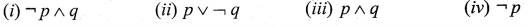Question 3.
Determine the truth value of each of the following statements
(i) If 6 + 2 = 5 , then the milk is white.
(ii) China is in Europe or $$\sqrt{3}$$ is an integer
(iii) It is not true that 5 + 5 = 9 or Earth is a planet
(iv) 11 is a prime number and all the sides of a rectangle are equal
Solution: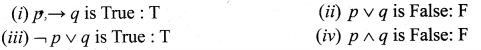Question 4.
Which one of the following sentences is a proposition?
(i) 4 + 7 = 12
(ii) What are you doing?
(iii) 3n ≤ 81, n ∈ N
(iv) Peacock is our national bird
(v) How tall this mountain is!
Solution:
(i) is a proposition
(ii) not a proposition
(iii) is a proposition
(iv) is a proposition
(v) not a proposition

Question 5.
Write the converse, inverse, and contrapositive of each of the following implication.
(i) If x and y are numbers such that x = y, then x2 = y2
(ii) If a quadrilateral is a square then it is a rectangle
Solution:
(i) Converse: If x and y are numbers such that x2 = y2 then x = y.
Inverse: If x and y are numbers such that x ≠ y then x2 ≠ y2.
Contrapositive : If x and v are numbers such that x2 ≠ y2 then x ≠ y.

(ii) Converse: If a quadrilateral is a rectangle then it is a square.
Inverse: If a quadrilateral is not a square then it is not a rectangle.
Contrapositive : If a quadrilateral is not a rectangle then it is not a square.Question 6.
Construct the truth table for the following statements.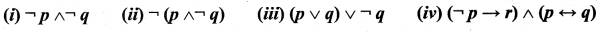Solution: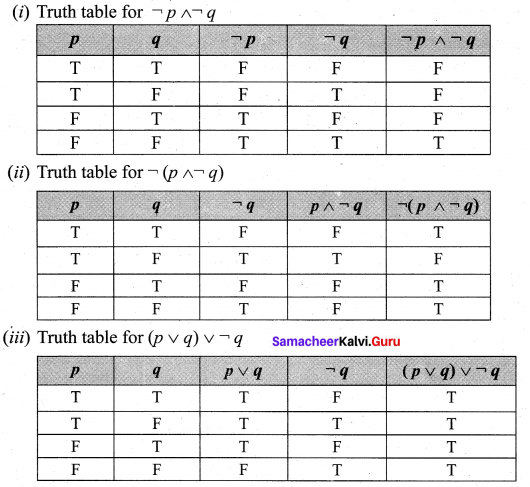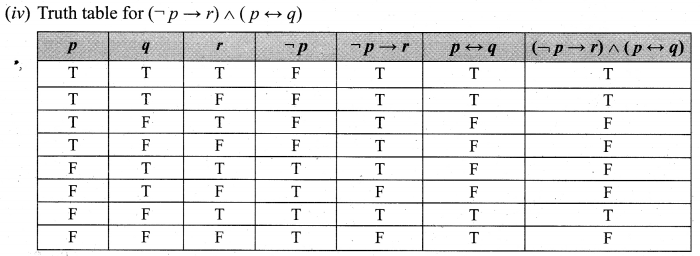Question 7.
Verify whether the following compound propositions are tautologies or contradictions or contingency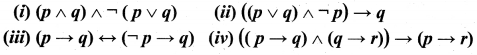Solution: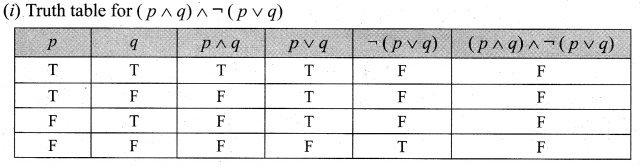In the above Truth table the last column entries are ‘F’. So the given propositions is a contradiction.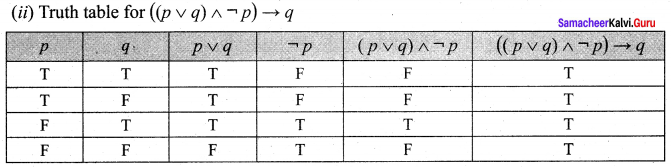In the above truth table the last column entries are ‘T’. So the given propositions is a tautology.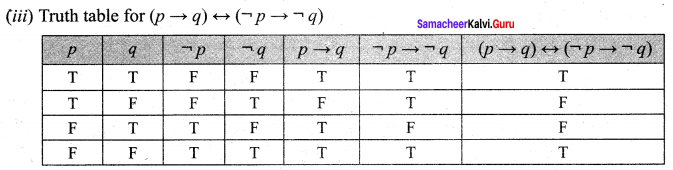In the above truth table the entries in the last column are a combination of’ T ‘ and ‘ F ‘. So the given statement is neither propositions is neither tautology nor a contradiction. It is a contingency.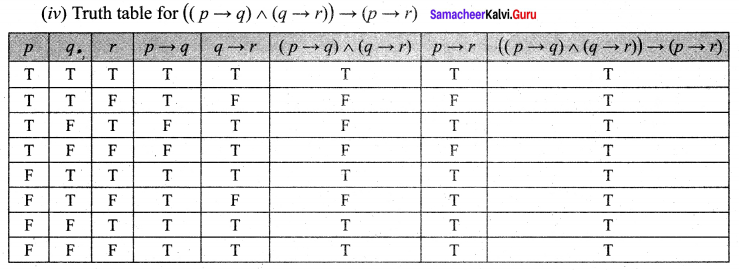The last column entires are ‘T’. So the given proposition is a tautology.Question 8.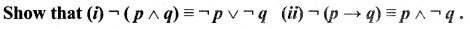Solution: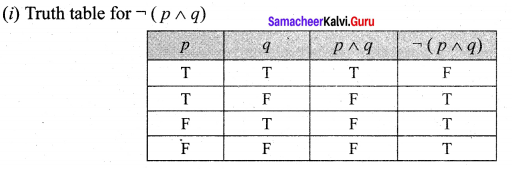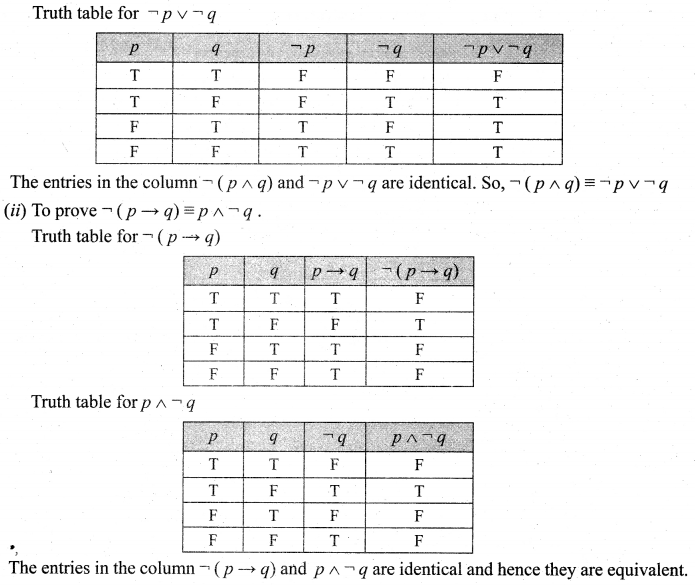Question 9.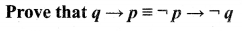Solution: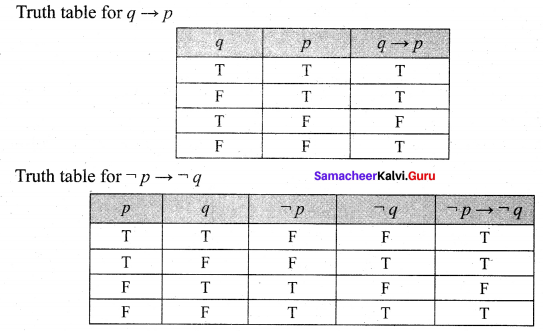The entries in the column corresponding to q ➝ p and $$\neg p \rightarrow \neg q$$ are identical and hence they are equivalent.

Question 10.
Show that p ➝ q and q ➝ p are not equivalent
Solution: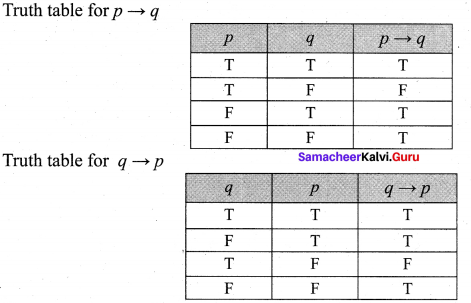The entries in the column corresponding to p ➝ q and q ➝ p are not identical, hence they are not equivalent.

Question 11.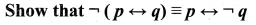Solution: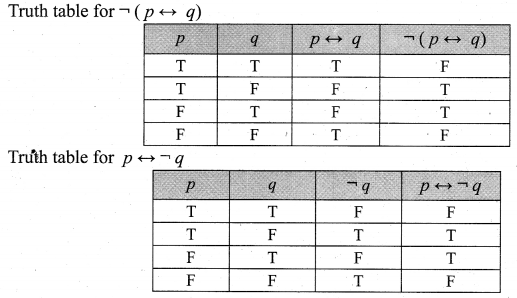Question 12.
Check whether the statement p ➝ (q ➝ p) is a tautology or a contradiction without using the truth table.
Solution: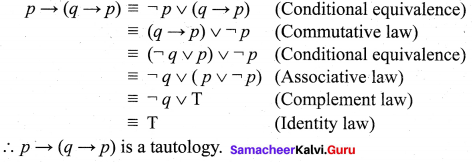Question 13.
Using truth table check whether the statements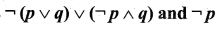are logically equivalent.
Solution: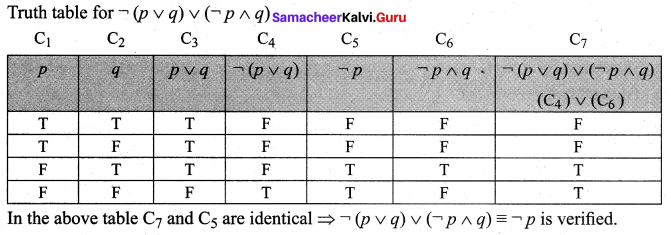Question 14.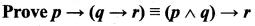without using truth table
Solution: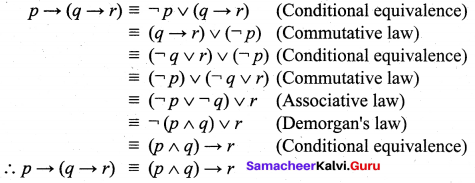Question 15.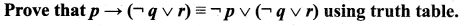Solution: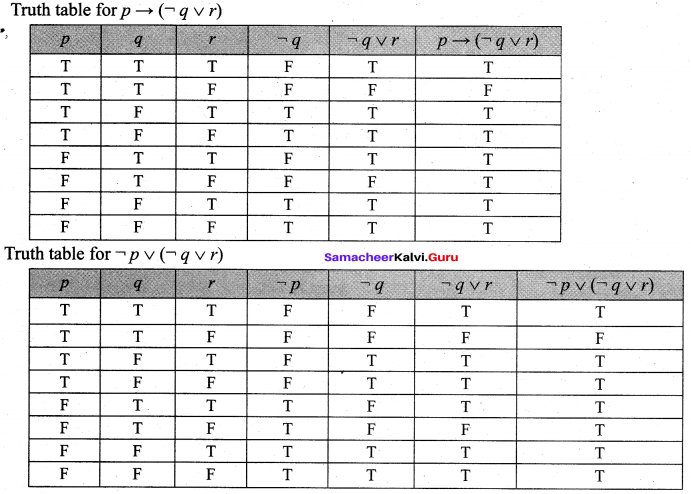The entries in the column corresponding to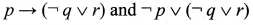are identical.
Hence they are equivalent.### Samacheer Kalvi 12th Maths Solutions Chapter 12 Discrete Mathematics Ex 12.2 Additional Problems

Question 1.
Show that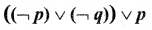is a tautology.
Solution: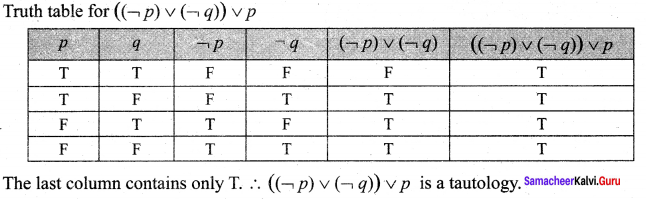Question 2.
Show that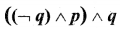is a contradiction.
Solution: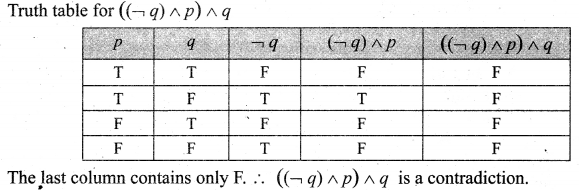Question 3.
Use the truth table to determine whether the statement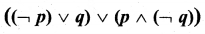is a tautology.
Solution: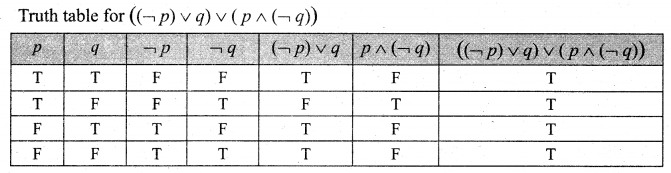The last column contains only T. ∴ The given statement is a tautology.

Question 4.
Show that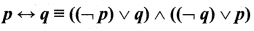Solution:
(i) Truth table for p $$\leftrightarrow$$ q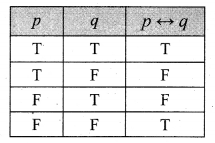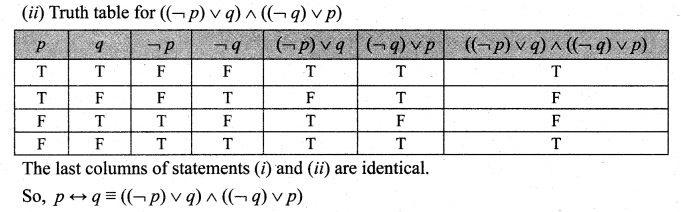Question 5.
Show that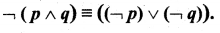.
Solution: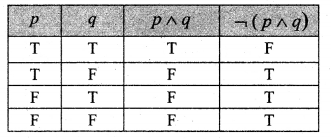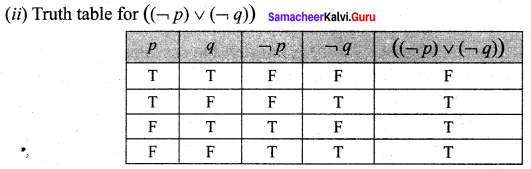The last columns of statements (i) and (ii) are identical.